# Flex Sensor Interfacing with AVR Microcontroller

Published  October 1, 2015   1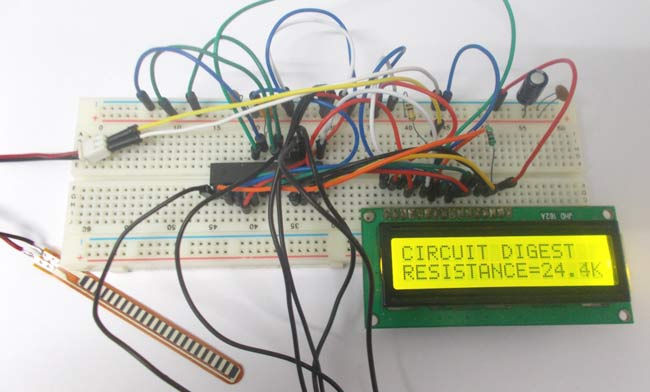In this tutorial we are going to interface FLEX sensor with ATMEGA8 microcontroller. In ATMEGA8, we are going use 10bit ADC (Analog to Digital Conversion) feature to do this job. Now the ADC in ATMEGA cannot take a input more than +5V.

### What is a Flex Sensor?

A FLEX sensor is a transducer which changes its resistance when its shape is changed.  It is shown in below figure.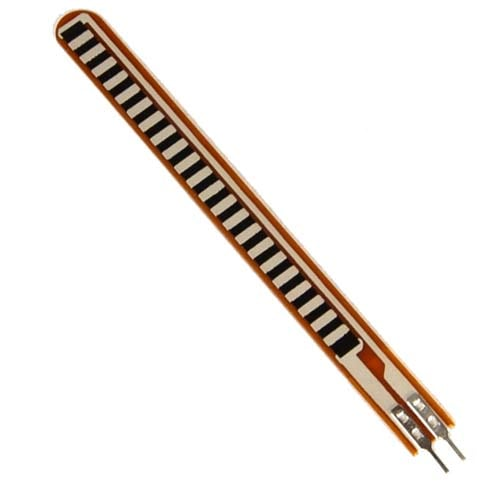This sensor is used to sense the changes in linearity. So when the FLEX sensor is bent the resistance is bent drastically. This is shown in below figure.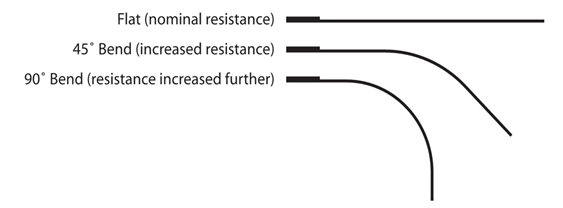Now for converting this change in resistance to change in voltage, we are going to use a voltage divider circuit. In this resistive network we have one constant resistance and other variable resistance. As shown in below figure, R1 here is a constant resistance and R2 is FLEX sensor which acts as a resistance. The midpoint of branch is taken to measurement. When resistance R2 changes,  the Vout changes with it linearly. So with this we have a voltage which changes with linearity.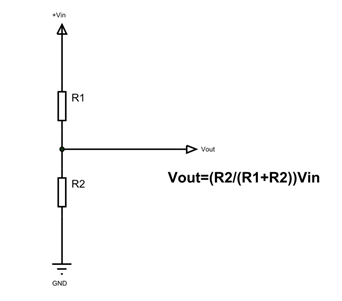Now important thing to note here is, the input taken by the controller for ADC conversion is as low as 50µAmp. This loading effect of resistance based voltage divider is important as the current drawn from Vout of voltage divider increases the error percentage, for now we need not worry about loading effect.

We are going take two resistors and form a divider circuit so that for a 25Volts Vin, we get a 5Volt Vout. So all we have to do is multiply the Vout value with “5” in the program in order to get the real input voltage.

### Components Required

HARDWARE: ATMEGA8, Power supply (5v), AVR-ISP PROGRAMMER, JHD_162ALCD (16x2LCD), 100uF capacitor, 100nF capacitor (5 pieces), 100KΩ resistor.

SOFTWARE: Atmel studio 6.1, progisp or flash magic.

### Circuit Diagram and Working Explanation

In the circuit PORTD of ATMEGA8 is connected to data port LCD. In 16x2 LCD there are 16 pins over all if there is a back light, if there is no back light there will be 14 pins. One can power or leave the back light pins. Now in the 14 pins there are 8 data pins (7-14 or D0-D7), 2 power supply pins (1&2 or VSS&VDD or gnd&+5v), 3rd pin for contrast control (VEE-controls how thick the characters should be shown) and 3 control pins (RS&RW&E).

In the circuit, you can observe that I have only took two control pins. The contrast bit and READ/WRITE are not often used so they can be shorted to ground. This puts LCD in highest contrast and read mode. We just need to control ENABLE and RS pins to send characters and data accordingly.

The LCD connections with ATmega8 are as follows:

PIN1 or VSS to Ground

PIN2 or VDD or VCC to +5v power

PIN3 or VEE to Ground (gives maximum contrast best for a beginner)

PIN4 or RS (Register Selection) to PB0 of uC

PIN5 or RW (Read/Write) to Ground (puts LCD in read mode eases the communication for user)

PIN6 or E (Enable) to PB1 of uC

PIN7 or D0 to PD0 of uC

PIN8 or D1 to PD1 of uC

PIN9 or D2 to PD2 of uC

PIN10 or D3 to PD3 of uC

PIN11 or D4 to D4 of uC

PIN12 or D5 to PD5 of uC

PIN13 or D6 to PD6 of uC

PIN14 or D7 to PD7 of uC

In the circuit you can see we have used 8bit communication (D0-D7) however this is not a compulsory, we can use 4bit communication (D4-D7) but with 4 bit communication program becomes a bit complex so we just went with 8 bit communication. (Also check this tutorial: 16x2 LCD interfacing with AVR microcontroller)

So from mere observation from above table we are connecting 10 pins of LCD to controller in which 8 pins are data pins and 2 pins are for control.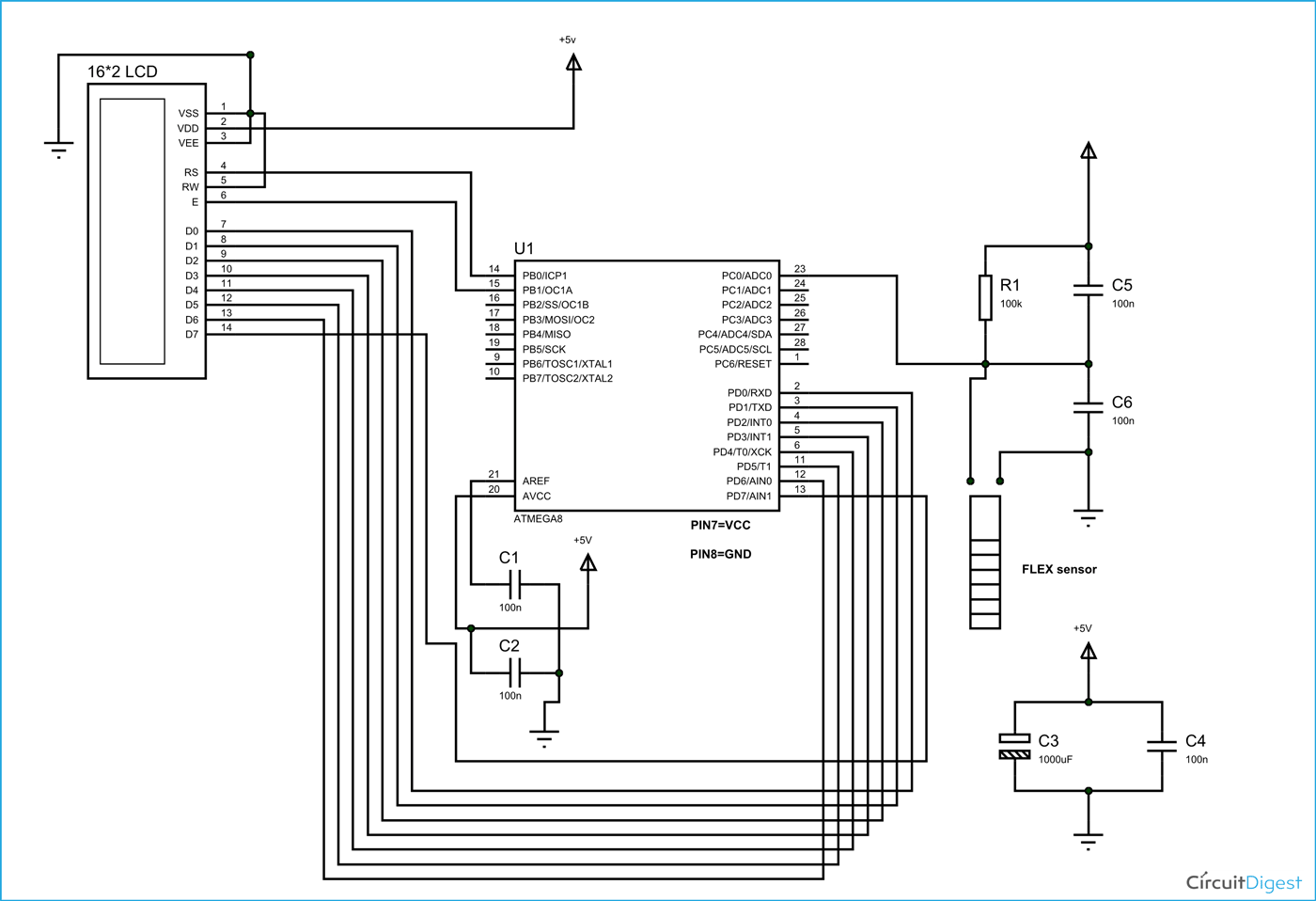The voltage across R2 is not completely linear; it will be a noisy one. To filter out the noise capacitors are placed across each resistor in the divider circuit as shown in figure.

In ATMEGA8, we can give Analog input to any of FOUR channels of PORTC, it doesn’t matter which channel we choose as all are same, we are going to choose channel 0 or PIN0 of PORTC.

In ATMEGA8, the ADC is of 10 bit resolution, so the controller can detect a sense a minimum change of Vref/2^10, so if the reference voltage is 5V we get a digital output increment for every 5/2^10 = 5mV. So for every 5mV increment in the input we will have a increment of one at digital output.

Now we need to set the register of ADC based on the following terms,

1. First of all we need to enable the ADC feature in ADC.

2. Here are going to get a maximum input voltage for ADC conversion is +5V. So we can set up maximum value or reference of ADC to 5V.

3. The controller has a trigger conversion feature that means ADC conversion takes place only after an external trigger, since we don’t want that we need to set the registers for the ADC to run in continuous free running mode.

4. For any ADC, frequency of conversion (Analog value to Digital value) and accuracy of digital output are inversely proportional. So for better accuracy of digital output we have to choose lesser frequency. For normal ADC clock we are setting the presale of ADC to maximum value (2). Since we are using the internal clock of 1MHZ, the clock of ADC will be (1000000/2).

These are the only four things we need to know to getting started with ADC.

All the above four features are set by two registers: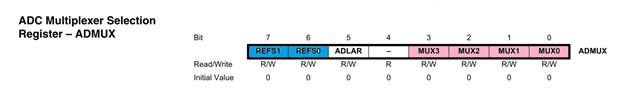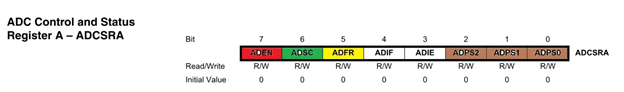RED (ADEN): This bit has to be set for enabling the ADC feature of ATMEGA.

BLUE(REFS1,REFS0): These two bits are used to set the reference voltage (or max input voltage we are going to give).  Since we want to have reference voltage 5V, REFS0 should be set, by the table.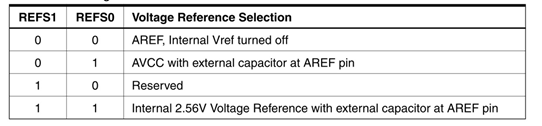YELLOW (ADFR): This bit must be set for the ADC to run continuously (free running mode).

PINK (MUX0-MUX3): These four bits are for telling the input channel. Since we are going to use ADC0 or PIN0, we need not set any bits as by the table.BROWN (ADPS0-ADPS2): these three bits are for setting the prescalar for ADC. Since we are using a prescalar of 2, we have to set one bit.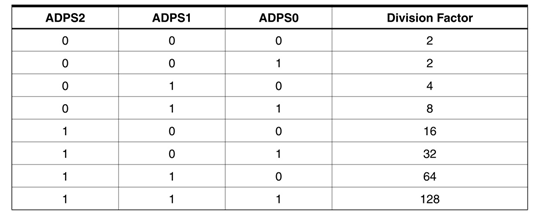DARK GREEN (ADSC): this bit set for the ADC to start conversion. This bit can be disabled in the program when we need to stop the conversion.

FLEX sensor interfacing with ATmega8 is explained step by step in C code given below.

Code

#include <avr/io.h>

//header to enable data flow control over pins

#define F_CPU 1000000

//telling controller crystal frequency attached

#include <util/delay.h>

//header to enable delay function in program

#define    E   5

//giving name “enable”  to 5th pin of PORTD, since it Is connected to LCD enable pin

#define RS  6

//giving name “registerselection” to 6th pin of PORTD, since is connected to LCD RS pin

void send_a_command(unsigned char command);

void send_a_character(unsigned char character);

void send_a_string(char *string_of_characters);

int main(void)

{

DDRB = 0xFF;

//putting portB and portD as output pins

DDRD = 0xFF;

_delay_ms(50);//giving delay of 50ms

DDRC = 0;//Taking portC as input.

//enabling the ADC, setting free running mode, setting prescalar 2

float i =0;

float RESISTANCE= 0;//storing digital output

char RESISTANCESHOW ;//displaying digital output as resistance in 16*2 lcd

send_a_command(0x01); //Clear Screen 0x01 = 00000001

_delay_ms(50);

send_a_command(0x38);//telling lcd we are using 8bit command /data mode

_delay_ms(50);

send_a_command(0b00001111);//LCD SCREEN ON and courser blinking

send_a_string ("CIRCUIT DIGEST  ");// displaying name

send_a_command(0x80 + 0x40 + 0);// shifting cursor  to 1st  shell  of second line

send_a_string ("RESISTANCE=");// displaying name

send_a_command(0x80 + 0x40 + 11);// shifting cursor  to 10th   shell  of second line

while(1)

{

i=ADC/204.8;//Now since it’s a 10bit ADC for every Vref(5V)/1024=5mV(4.88mV) we get one digital increment or for every 1V increment in input we get 204.8 count increment. So for finding voltage at ADC pin.

dtostrf(RESISTANCE, 4, 1, RESISTANCESHOW);

send_a_string(RESISTANCESHOW);

send_a_string("K");                        //dtostr(double precision value, width, precision, string that will store the numbers);

// Value is either a direct value plugged into this place, or a variable to contains a value.

//Width that is used with dtostrf is the number of characters in the number that includes the negative sign (-). For instance, if the number is -532.87, the width would be 7 including the negative sign and the decimal point.

//Precision is how many numbers would be after the decimal point in the dtostrf usage.

_delay_ms(50);

send_a_command(0x80 + 0x40 + 11);//retuning to second line 10th shell.

}

}

void send_a_command(unsigned char command)

{

PORTA = command;

PORTD &= ~ (1<<RS); //putting 0 in RS to tell lcd we are sending command

PORTD |= 1<<E; //telling lcd to receive command /data at the port

_delay_ms(50);

PORTD &= ~1<<E;//telling lcd we completed sending data

PORTA= 0;

}

void send_a_character(unsigned char character)

{

PORTA= character;

PORTD |= 1<<RS;//telling LCD we are sending data not commands

PORTD |= 1<<E;//telling LCD to start receiving command/data

_delay_ms(50);

PORTD &= ~1<<E;//telling lcd we completed sending data/command

PORTA = 0;

}

void send_a_string(char *string_of_characters)

{

while(*string_of_characters > 0)

{

send_a_character(*string_of_characters++);

}

}

Video

Tags